## Set Up Multivariate Regression Problems

### Response Matrix

To fit a multivariate linear regression model using `mvregress`, you must set up your response matrix and design matrices in a particular way. Given properly formatted inputs, `mvregress` can handle a variety of multivariate regression problems.

`mvregress` expects the n observations of potentially correlated d-dimensional responses to be in an n-by-d matrix, named `Y`, for example. That is, set up your responses so that the dependency structure is between observations in the same row. If you specify `Y` as a vector of length n (either a row or column vector), then `mvregress` assumes that d = 1, and treats the elements as n independent observations. It does not model the vector as one realization of a correlated series (such as a time series).

To illustrate how to set up a response matrix, suppose that your multivariate responses are repeated measurements made on subjects at multiple time points, as in the following figure.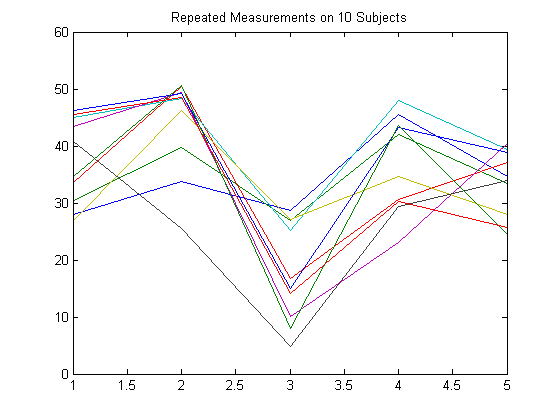Suppose that observations within a subject are correlated.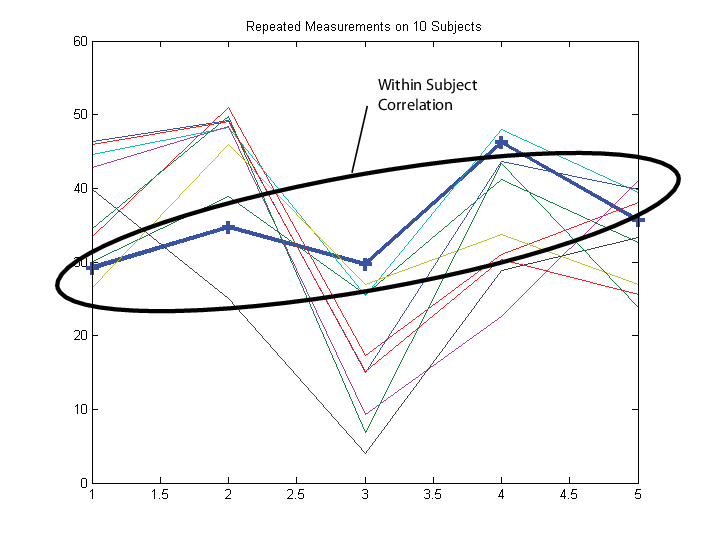In this case, set up the response matrix `Y` such that each row corresponds to a subject, and each column corresponds to a time point.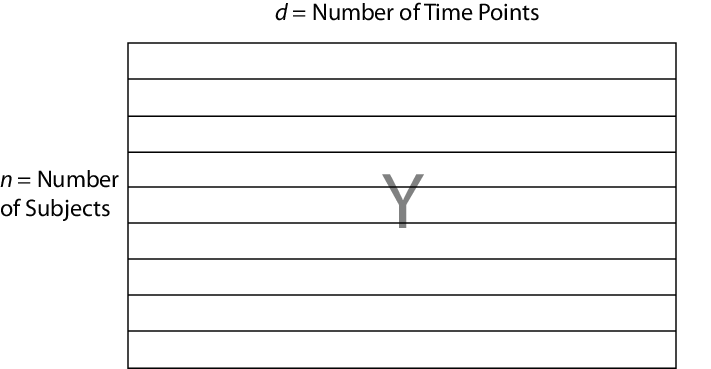Then again, suppose that observations made on subjects at the same time are correlated (concurrent correlation).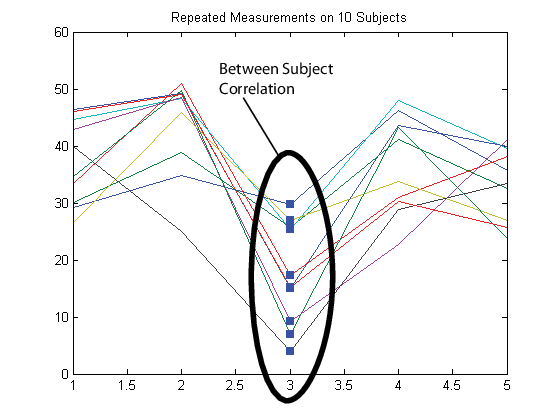In this case, set up the response matrix `Y` such that each row corresponds to a time point, and each column corresponds to a subject.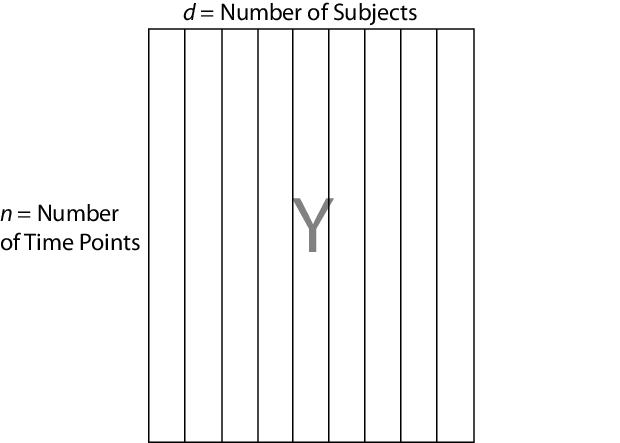### Design Matrices

In the multivariate linear regression model, each d-dimensional response has a corresponding design matrix. Depending on the model, the design matrix might be comprised of exogenous predictor variables, dummy variables, lagged responses, or a combination of these and other covariate terms.

• If d > 1 and all d dimensions have the same design matrix, then specify one n-by-p design matrix, where p is the number of predictor variables. To determine an intercept for each dimension, add a column of ones to the design matrix. In this case, `mvregress` applies the design matrix to all d dimensions.

• If d > 1 and all d dimensions do not have the same design matrix, then specify the design matrices using a length-n cell array of d-by-K arrays, named `X`, for example. K is the total number of regression coefficients in the model. Note that the rows of the arrays in `X` correspond to the columns of the response matrix, `Y`.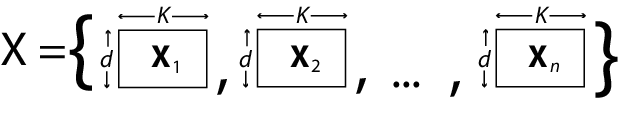If all n observations have the same design matrix, you can specify a cell array containing one d-by-K design matrix. In this case, `mvregress` applies the design matrix to all n observations. For example, this situation might arise if the predictors are functions of time, and all observations were measured at the same time points.

• In the special case that d = 1, you can specify one n-by-K design matrix (not in a cell array). However, you should consider using `fitlm` to fit regression models to univariate, continuous responses.

The following sections illustrate how to set up the some common multivariate regression problems for estimation using `mvregress`.

### Common Multivariate Regression Problems

#### Multivariate General Linear Model

The multivariate general linear model is of the form

`${Y}_{n×d}={X}_{n×\left(p+1\right)}{B}_{\left(p+1\right)×d}+{E}_{n×d}.$`

In expanded form,

`$\left[\begin{array}{l}{y}_{11}\text{\hspace{0.17em}}\text{\hspace{0.17em}}\text{\hspace{0.17em}}\text{\hspace{0.17em}}{y}_{12}\text{\hspace{0.17em}}\text{\hspace{0.17em}}\text{\hspace{0.17em}}\text{\hspace{0.17em}}\cdots \text{\hspace{0.17em}}\text{\hspace{0.17em}}\text{\hspace{0.17em}}\text{\hspace{0.17em}}{y}_{1d}\\ {y}_{21}\text{\hspace{0.17em}}\text{\hspace{0.17em}}\text{\hspace{0.17em}}\text{\hspace{0.17em}}{y}_{22}\text{\hspace{0.17em}}\text{\hspace{0.17em}}\text{\hspace{0.17em}}\text{\hspace{0.17em}}\cdots \text{\hspace{0.17em}}\text{\hspace{0.17em}}\text{\hspace{0.17em}}\text{\hspace{0.17em}}{y}_{2d}\\ \text{\hspace{0.17em}}\text{\hspace{0.17em}}\text{\hspace{0.17em}}⋮\text{\hspace{0.17em}}\text{\hspace{0.17em}}\text{\hspace{0.17em}}\text{\hspace{0.17em}}\text{\hspace{0.17em}}\text{\hspace{0.17em}}\text{\hspace{0.17em}}\text{\hspace{0.17em}}\text{\hspace{0.17em}}\text{\hspace{0.17em}}\text{\hspace{0.17em}}\text{\hspace{0.17em}}\text{\hspace{0.17em}}⋮\text{\hspace{0.17em}}\text{\hspace{0.17em}}\text{\hspace{0.17em}}\text{\hspace{0.17em}}\text{\hspace{0.17em}}\text{\hspace{0.17em}}\text{\hspace{0.17em}}\text{\hspace{0.17em}}\ddots \text{\hspace{0.17em}}\text{\hspace{0.17em}}\text{\hspace{0.17em}}\text{\hspace{0.17em}}\text{\hspace{0.17em}}⋮\text{\hspace{0.17em}}\text{\hspace{0.17em}}\text{\hspace{0.17em}}\text{\hspace{0.17em}}\\ {y}_{n1}\text{\hspace{0.17em}}\text{\hspace{0.17em}}\text{\hspace{0.17em}}\text{\hspace{0.17em}}{y}_{n2}\text{\hspace{0.17em}}\text{\hspace{0.17em}}\text{\hspace{0.17em}}\text{\hspace{0.17em}}\cdots \text{\hspace{0.17em}}\text{\hspace{0.17em}}\text{\hspace{0.17em}}{y}_{nd}\end{array}\right]=\left[\begin{array}{l}1\text{\hspace{0.17em}}\text{\hspace{0.17em}}\text{\hspace{0.17em}}\text{\hspace{0.17em}}{x}_{11}\text{\hspace{0.17em}}\text{\hspace{0.17em}}\text{\hspace{0.17em}}\text{\hspace{0.17em}}{x}_{12}\text{\hspace{0.17em}}\text{\hspace{0.17em}}\text{\hspace{0.17em}}\text{\hspace{0.17em}}\text{\hspace{0.17em}}\cdots \text{\hspace{0.17em}}\text{\hspace{0.17em}}\text{\hspace{0.17em}}\text{\hspace{0.17em}}{x}_{1p}\\ 1\text{\hspace{0.17em}}\text{\hspace{0.17em}}\text{\hspace{0.17em}}\text{\hspace{0.17em}}{x}_{21}\text{\hspace{0.17em}}\text{\hspace{0.17em}}\text{\hspace{0.17em}}\text{\hspace{0.17em}}{x}_{22}\text{\hspace{0.17em}}\text{\hspace{0.17em}}\text{\hspace{0.17em}}\text{\hspace{0.17em}}\cdots \text{\hspace{0.17em}}\text{\hspace{0.17em}}\text{\hspace{0.17em}}\text{\hspace{0.17em}}\text{\hspace{0.17em}}{x}_{2p}\\ ⋮\text{\hspace{0.17em}}\text{\hspace{0.17em}}\text{\hspace{0.17em}}\text{\hspace{0.17em}}\text{\hspace{0.17em}}\text{\hspace{0.17em}}\text{\hspace{0.17em}}\text{\hspace{0.17em}}\text{\hspace{0.17em}}⋮\text{\hspace{0.17em}}\text{\hspace{0.17em}}\text{\hspace{0.17em}}\text{\hspace{0.17em}}\text{\hspace{0.17em}}\text{\hspace{0.17em}}\text{\hspace{0.17em}}\text{\hspace{0.17em}}\text{\hspace{0.17em}}\text{\hspace{0.17em}}\text{\hspace{0.17em}}⋮\text{\hspace{0.17em}}\text{\hspace{0.17em}}\text{\hspace{0.17em}}\text{\hspace{0.17em}}\text{\hspace{0.17em}}\text{\hspace{0.17em}}\text{\hspace{0.17em}}\text{\hspace{0.17em}}\ddots \text{\hspace{0.17em}}\text{\hspace{0.17em}}\text{\hspace{0.17em}}\text{\hspace{0.17em}}\text{\hspace{0.17em}}\text{\hspace{0.17em}}\text{\hspace{0.17em}}\text{\hspace{0.17em}}\text{\hspace{0.17em}}⋮\\ 1\text{\hspace{0.17em}}\text{\hspace{0.17em}}\text{\hspace{0.17em}}\text{\hspace{0.17em}}\text{\hspace{0.17em}}{x}_{n1}\text{\hspace{0.17em}}\text{\hspace{0.17em}}\text{\hspace{0.17em}}{x}_{n2}\text{\hspace{0.17em}}\text{\hspace{0.17em}}\text{\hspace{0.17em}}\cdots \text{\hspace{0.17em}}\text{\hspace{0.17em}}\text{\hspace{0.17em}}\text{\hspace{0.17em}}{x}_{np}\end{array}\right]\left[\begin{array}{l}{\beta }_{01}\text{\hspace{0.17em}}\text{\hspace{0.17em}}\text{\hspace{0.17em}}\text{\hspace{0.17em}}\text{\hspace{0.17em}}\text{\hspace{0.17em}}\text{\hspace{0.17em}}{\beta }_{02}\text{\hspace{0.17em}}\text{\hspace{0.17em}}\text{\hspace{0.17em}}\text{\hspace{0.17em}}\cdots \text{\hspace{0.17em}}\text{\hspace{0.17em}}\text{\hspace{0.17em}}\text{\hspace{0.17em}}{\beta }_{0d}\\ {\beta }_{11}\text{\hspace{0.17em}}\text{\hspace{0.17em}}\text{\hspace{0.17em}}\text{\hspace{0.17em}}\text{\hspace{0.17em}}\text{\hspace{0.17em}}\text{\hspace{0.17em}}{\beta }_{12}\text{\hspace{0.17em}}\text{\hspace{0.17em}}\text{\hspace{0.17em}}\text{\hspace{0.17em}}\text{\hspace{0.17em}}\cdots \text{\hspace{0.17em}}\text{\hspace{0.17em}}\text{\hspace{0.17em}}\text{\hspace{0.17em}}{\beta }_{1d}\\ \text{\hspace{0.17em}}\text{\hspace{0.17em}}\text{\hspace{0.17em}}\text{\hspace{0.17em}}⋮\text{\hspace{0.17em}}\text{\hspace{0.17em}}\text{\hspace{0.17em}}\text{\hspace{0.17em}}\text{\hspace{0.17em}}\text{\hspace{0.17em}}\text{\hspace{0.17em}}\text{\hspace{0.17em}}\text{\hspace{0.17em}}\text{\hspace{0.17em}}\text{\hspace{0.17em}}\text{\hspace{0.17em}}\text{\hspace{0.17em}}\text{\hspace{0.17em}}⋮\text{\hspace{0.17em}}\text{\hspace{0.17em}}\text{\hspace{0.17em}}\text{\hspace{0.17em}}\text{\hspace{0.17em}}\text{\hspace{0.17em}}\text{\hspace{0.17em}}\text{\hspace{0.17em}}\text{\hspace{0.17em}}\ddots \text{\hspace{0.17em}}\text{\hspace{0.17em}}\text{\hspace{0.17em}}\text{\hspace{0.17em}}\text{\hspace{0.17em}}\text{\hspace{0.17em}}\text{\hspace{0.17em}}\text{\hspace{0.17em}}\text{\hspace{0.17em}}⋮\\ {\beta }_{p1}\text{\hspace{0.17em}}\text{\hspace{0.17em}}\text{\hspace{0.17em}}\text{\hspace{0.17em}}\text{\hspace{0.17em}}{\beta }_{p2}\text{\hspace{0.17em}}\text{\hspace{0.17em}}\text{\hspace{0.17em}}\text{\hspace{0.17em}}\text{\hspace{0.17em}}\cdots \text{\hspace{0.17em}}\text{\hspace{0.17em}}\text{\hspace{0.17em}}{\beta }_{pd}\end{array}\right]+\left[\begin{array}{l}{\epsilon }_{11}\text{\hspace{0.17em}}\text{\hspace{0.17em}}\text{\hspace{0.17em}}\text{\hspace{0.17em}}{\epsilon }_{12}\text{\hspace{0.17em}}\text{\hspace{0.17em}}\text{\hspace{0.17em}}\text{\hspace{0.17em}}\cdots \text{\hspace{0.17em}}\text{\hspace{0.17em}}\text{\hspace{0.17em}}\text{\hspace{0.17em}}{\epsilon }_{1d}\\ {\epsilon }_{21}\text{\hspace{0.17em}}\text{\hspace{0.17em}}\text{\hspace{0.17em}}\text{\hspace{0.17em}}{\epsilon }_{22}\text{\hspace{0.17em}}\text{\hspace{0.17em}}\text{\hspace{0.17em}}\text{\hspace{0.17em}}\cdots \text{\hspace{0.17em}}\text{\hspace{0.17em}}\text{\hspace{0.17em}}\text{\hspace{0.17em}}{\epsilon }_{2d}\\ \text{\hspace{0.17em}}\text{\hspace{0.17em}}\text{\hspace{0.17em}}⋮\text{\hspace{0.17em}}\text{\hspace{0.17em}}\text{\hspace{0.17em}}\text{\hspace{0.17em}}\text{\hspace{0.17em}}\text{\hspace{0.17em}}\text{\hspace{0.17em}}\text{\hspace{0.17em}}\text{\hspace{0.17em}}\text{\hspace{0.17em}}\text{\hspace{0.17em}}\text{\hspace{0.17em}}\text{\hspace{0.17em}}⋮\text{\hspace{0.17em}}\text{\hspace{0.17em}}\text{\hspace{0.17em}}\text{\hspace{0.17em}}\text{\hspace{0.17em}}\text{\hspace{0.17em}}\text{\hspace{0.17em}}\text{\hspace{0.17em}}\ddots \text{\hspace{0.17em}}\text{\hspace{0.17em}}\text{\hspace{0.17em}}\text{\hspace{0.17em}}\text{\hspace{0.17em}}⋮\text{\hspace{0.17em}}\text{\hspace{0.17em}}\text{\hspace{0.17em}}\text{\hspace{0.17em}}\\ {\epsilon }_{n1}\text{\hspace{0.17em}}\text{\hspace{0.17em}}\text{\hspace{0.17em}}\text{\hspace{0.17em}}{\epsilon }_{n2}\text{\hspace{0.17em}}\text{\hspace{0.17em}}\text{\hspace{0.17em}}\text{\hspace{0.17em}}\cdots \text{\hspace{0.17em}}\text{\hspace{0.17em}}\text{\hspace{0.17em}}{\epsilon }_{nd}\end{array}\right].$`

That is, each d-dimensional response has an intercept and p predictor variables, and each dimension has its own set of regression coefficients. In this form, the least squares solution is `B = X\Y`. To estimate this model using `mvregress`, use the n-by-d matrix of responses, as above.

If all d dimensions have the same design matrix, use the n-by-(p+1) design matrix, as above. Adding a column of ones to the p predictor variables computes the intercept for each dimension.

If all d dimensions do not have the same design matrix, reformat the n-by-(p + 1) design matrix into a length-n cell array of d-by-K matrices. Here, K = (p + 1)d for an intercept and slopes for each dimension.

For example, suppose n = 4, d = 3, and p = 2 (two predictor terms in addition to an intercept). This figure shows how to format the ith element in the cell array.

`$\left[\begin{array}{ccc}{y}_{11}& {y}_{12}& {y}_{13}\\ \begin{array}{c}{y}_{21}\\ {y}_{31}\end{array}& \begin{array}{c}{y}_{22}\\ {y}_{32}\end{array}& \begin{array}{c}{y}_{23}\\ {y}_{33}\end{array}\\ {y}_{41}& {y}_{42}& {y}_{43}\end{array}\right]=\underset{\begin{array}{c}↓\\ \underset{X\left\{i\right\}}{\underbrace{\left[\begin{array}{ccc}\begin{array}{ccc}1& 0& 0\\ 0& 1& 0\\ 0& 0& 1\end{array}& \begin{array}{ccc}{x}_{i1}& 0& 0\\ 0& {x}_{i1}& 0\\ 0& 0& {x}_{i1}\end{array}& \begin{array}{ccc}{x}_{i2}& 0& 0\\ 0& {x}_{i2}& 0\\ 0& 0& {x}_{i2}\end{array}\end{array}\right]}}\left[\begin{array}{c}{\beta }_{01}\\ {\beta }_{02}\\ {\beta }_{03}\\ \begin{array}{c}\begin{array}{c}{\beta }_{11}\\ {\beta }_{12}\\ {\beta }_{13}\end{array}\\ {\beta }_{21}\\ {\beta }_{22}\\ {\beta }_{23}\end{array}\end{array}\right]\end{array}}{\underbrace{\left[\begin{array}{ccc}1& {x}_{11}& {x}_{12}\\ \begin{array}{c}1\\ 1\end{array}& \begin{array}{c}{x}_{21}\\ {x}_{31}\end{array}& \begin{array}{c}{x}_{22}\\ {x}_{32}\end{array}\\ 1& {x}_{41}& {x}_{42}\end{array}\right]\left[\begin{array}{ccc}{\beta }_{01}& {\beta }_{02}& {\beta }_{03}\\ {\beta }_{11}& {\beta }_{12}& {\beta }_{13}\\ {\beta }_{21}& {\beta }_{22}& {\beta }_{23}\end{array}\right]}}+\left[\begin{array}{ccc}{\epsilon }_{11}& {\epsilon }_{12}& {\epsilon }_{13}\\ \begin{array}{c}{\epsilon }_{21}\\ {\epsilon }_{31}\end{array}& \begin{array}{c}{\epsilon }_{22}\\ {\epsilon }_{32}\end{array}& \begin{array}{c}{\epsilon }_{23}\\ {\epsilon }_{33}\end{array}\\ {\epsilon }_{41}& {\epsilon }_{42}& {\epsilon }_{43}\end{array}\right]$`

If you prefer, you can reshape the K-by-1 vector of coefficients back into a (p + 1)-by-d matrix after estimation.

To put constraints on the model parameters, adjust the design matrix accordingly. For example, suppose that the three dimensions in the previous example have a common slope. That is, ${\beta }_{11}={\beta }_{12}={\beta }_{13}={\beta }_{1}$ and ${\beta }_{21}={\beta }_{22}={\beta }_{23}={\beta }_{2}.$ In this case, each design matrix is 3-by-5, as shown in the following figure.

`$\underset{X\left\{i\right\}}{\underbrace{\left[\begin{array}{ccc}\begin{array}{ccc}1& 0& 0\end{array}& {x}_{i1}& {x}_{i2}\\ \begin{array}{ccc}0& 1& 0\end{array}& {x}_{i1}& {x}_{i2}\\ \begin{array}{ccc}0& 0& 1\end{array}& {x}_{i1}& {x}_{i2}\end{array}\right]}}\left[\begin{array}{c}\begin{array}{c}{\beta }_{01}\\ {\beta }_{02}\end{array}\\ {\beta }_{03}\\ {\beta }_{1}\\ {\beta }_{2}\end{array}\right]$`

#### Longitudinal Analysis

In a longitudinal analysis, you might measure responses on n subjects at d time points, with correlation between observations made on the same subject. For example, suppose that you measure responses yij at times tij, i = 1,...,n and j = 1,...,d. In addition, suppose that each subject is in one of two groups (such as male or female), specified by the indicator variable Gi. You could model yij as a function of Gi and tij, with group-specific intercepts and slopes, as follows:

`${y}_{ij}={\beta }_{0}+{\beta }_{1}{G}_{i}+{\beta }_{2}{t}_{ij}+{\beta }_{3}{G}_{i}×{t}_{ij}+{\epsilon }_{ij},\text{\hspace{0.17em}}\text{\hspace{0.17em}}i=1,\dots ,n;\text{\hspace{0.17em}}j=1,\dots ,d,$`

where

`${\epsilon }_{i}=\left({\epsilon }_{i1},\dots ,{\epsilon }_{id}{\right)}^{\prime }\sim MVN\left(0,\Sigma \right).$`

Most longitudinal models include time as an explicit predictor.

To fit this model using `mvregress`, arrange the responses in an n-by-d matrix, where n is the number of subjects and d is the number of time points. Specify the design matrices in an n-length cell array of d-by-K matrices, where here K = 4 for the four regression coefficients.

For example, suppose d = 5 (five observations per subject). The ith design matrix and corresponding parameter vector for the specified model are shown in the following figure.

`$\underset{X\left\{i\right\}}{\underbrace{\left[\begin{array}{cccc}1& {G}_{i}& {t}_{i1}& {G}_{i}×{t}_{i1}\\ 1& {G}_{i}& {t}_{i2}& {G}_{i}×{t}_{i2}\\ 1& {G}_{i}& {t}_{i3}& {G}_{i}×{t}_{i3}\\ \begin{array}{c}1\\ 1\end{array}& \begin{array}{c}{G}_{i}\\ {G}_{i}\end{array}& \begin{array}{c}{t}_{i4}\\ {t}_{i5}\end{array}& \begin{array}{c}{G}_{i}×{t}_{i4}\\ {G}_{i}×{t}_{i5}\end{array}\end{array}\right]}}\left[\begin{array}{c}{\beta }_{0}\\ {\beta }_{1}\\ {\beta }_{2}\\ {\beta }_{3}\end{array}\right]$`

#### Panel Analysis

In a panel analysis, you might measure responses and covariates on d subjects (such as individuals or countries) at n time points. For example, suppose you measure responses ytj and covariates xtj on subjects j = 1,...,d at times t = 1,...,n. A fixed effects panel model, with subject-specific fixed effects, and concurrent correlation might look like:

`${y}_{tj}={\alpha }_{j}+\beta {x}_{tj}+{\epsilon }_{tj},$`

where

`${\epsilon }_{t}=\left({\epsilon }_{t1},...,{\epsilon }_{td}{\right)}^{\prime }\sim MVN\left(0,\Sigma \right).$`

In contrast to longitudinal models, the panel analysis model typically includes covariates measured at each time point, instead of using time as an explicit predictor.

To fit this model using `mvregress`, arrange the responses in an n-by-d matrix, such that each column corresponds to a subject. Specify the design matrices in an n-length cell array of d-by-K matrices, where here K = d + 1 for the d intercepts and a slope term.

For example, suppose d = 4 (four subjects). The tth design matrix and corresponding parameter vector are shown in the following figure.

`$\underset{X\left\{t\right\}}{\underbrace{\left[\begin{array}{cccc}1& 0& 0& \begin{array}{cc}0& {x}_{t1}\end{array}\\ 0& 1& 0& \begin{array}{cc}0& {x}_{t2}\end{array}\\ 0& 0& 1& \begin{array}{cc}0& {x}_{t3}\end{array}\\ 0& 0& 0& \begin{array}{cc}1& {x}_{t4}\end{array}\end{array}\right]}}\left[\begin{array}{c}\begin{array}{c}{\alpha }_{1}\\ {\alpha }_{2}\end{array}\\ {\alpha }_{3}\\ {\alpha }_{4}\\ \beta \end{array}\right]$`

#### Seemingly Unrelated Regression

In a seemingly unrelated regression (SUR), you model d separate regressions, each with its own intercept and slope, but a common error variance-covariance matrix. For example, suppose you measure responses yij and covariates xij for regression models j = 1,...,d, with i = 1,...,n observations to fit each regression. The SUR model might look like:

`${y}_{ij}={\beta }_{0j}+{\beta }_{j}{x}_{ij}+{\epsilon }_{ij},$`

where

`${\epsilon }_{i}=\left({\epsilon }_{i1},\dots ,{\epsilon }_{id}{\right)}^{\prime }\sim MVN\left(0,\Sigma \right).$`

This model is very similar to the multivariate general linear model, except that it has different covariates for each dimension.

To fit this model using `mvregress`, arrange the responses in an n-by-d matrix, such that each column has the data for the jth regression model. Specify the design matrices in an n-length cell array of d-by-K matrices, where here K = 2d for d intercepts and d slopes.

For example, suppose d = 3 (three regressions). The ith design matrix and corresponding parameter vector are shown in the following figure.

`$\underset{X\left\{i\right\}}{\underbrace{\left[\begin{array}{cc}\begin{array}{ccc}1& 0& 0\\ 0& 1& 0\\ 0& 0& 1\end{array}& \begin{array}{ccc}{x}_{i1}& 0& 0\\ 0& {x}_{i2}& 0\\ 0& 0& {x}_{i3}\end{array}\end{array}\right]}}\left[\begin{array}{c}\begin{array}{c}{\beta }_{01}\\ {\beta }_{02}\end{array}\\ {\beta }_{03}\\ {\beta }_{1}\\ \begin{array}{c}{\beta }_{2}\\ {\beta }_{3}\end{array}\end{array}\right]$`

#### Vector Autoregressive Model

The VAR(p) vector autoregressive model expresses d-dimensional time series responses as a linear function of p lagged d-dimensional responses from previous times. For example, suppose you measure responses ytj for time series j = 1,...,d at times t = 1,...,n. The VAR(p) model might look like:

`$\left[\begin{array}{l}{y}_{t1}\\ {y}_{t2}\\ \text{\hspace{0.17em}}\text{\hspace{0.17em}}\text{\hspace{0.17em}}⋮\\ {y}_{td}\end{array}\right]=\left[\begin{array}{l}{c}_{1}\\ {c}_{2}\\ \text{\hspace{0.17em}}\text{\hspace{0.17em}}⋮\\ {c}_{d}\end{array}\right]+\left[\begin{array}{l}{\phi }_{11}^{\left(1\right)}\text{\hspace{0.17em}}\text{\hspace{0.17em}}\text{\hspace{0.17em}}{\phi }_{12}^{\left(1\right)}\text{\hspace{0.17em}}\text{\hspace{0.17em}}\cdots \text{\hspace{0.17em}}\text{\hspace{0.17em}}{\phi }_{1d}^{\left(1\right)}\\ \text{\hspace{0.17em}}\text{\hspace{0.17em}}\text{\hspace{0.17em}}⋮\text{\hspace{0.17em}}\text{\hspace{0.17em}}\text{\hspace{0.17em}}\text{\hspace{0.17em}}\text{\hspace{0.17em}}\text{\hspace{0.17em}}\text{\hspace{0.17em}}\text{\hspace{0.17em}}\text{\hspace{0.17em}}⋮\text{\hspace{0.17em}}\text{\hspace{0.17em}}\text{\hspace{0.17em}}\text{\hspace{0.17em}}\text{\hspace{0.17em}}\text{\hspace{0.17em}}\ddots \text{\hspace{0.17em}}\text{\hspace{0.17em}}\text{\hspace{0.17em}}\text{\hspace{0.17em}}\text{\hspace{0.17em}}⋮\\ {\phi }_{d1}^{\left(1\right)}\text{\hspace{0.17em}}\text{\hspace{0.17em}}\text{\hspace{0.17em}}\text{\hspace{0.17em}}{\phi }_{d2}^{\left(1\right)}\text{\hspace{0.17em}}\text{\hspace{0.17em}}\cdots \text{\hspace{0.17em}}\text{\hspace{0.17em}}{\phi }_{dd}^{\left(1\right)}\end{array}\right]\left[\begin{array}{l}{y}_{t-1,1}\\ {y}_{t-1,2}\\ \text{\hspace{0.17em}}\text{\hspace{0.17em}}\text{\hspace{0.17em}}⋮\\ {y}_{t-1,d}\end{array}\right]+\cdots +\left[\begin{array}{l}{\phi }_{11}^{\left(p\right)}\text{\hspace{0.17em}}\text{\hspace{0.17em}}\text{\hspace{0.17em}}{\phi }_{12}^{\left(p\right)}\text{\hspace{0.17em}}\text{\hspace{0.17em}}\cdots \text{\hspace{0.17em}}\text{\hspace{0.17em}}{\phi }_{1d}^{\left(p\right)}\\ \text{\hspace{0.17em}}\text{\hspace{0.17em}}\text{\hspace{0.17em}}⋮\text{\hspace{0.17em}}\text{\hspace{0.17em}}\text{\hspace{0.17em}}\text{\hspace{0.17em}}\text{\hspace{0.17em}}\text{\hspace{0.17em}}\text{\hspace{0.17em}}\text{\hspace{0.17em}}\text{\hspace{0.17em}}⋮\text{\hspace{0.17em}}\text{\hspace{0.17em}}\text{\hspace{0.17em}}\text{\hspace{0.17em}}\text{\hspace{0.17em}}\text{\hspace{0.17em}}\ddots \text{\hspace{0.17em}}\text{\hspace{0.17em}}\text{\hspace{0.17em}}\text{\hspace{0.17em}}\text{\hspace{0.17em}}⋮\\ {\phi }_{d1}^{\left(p\right)}\text{\hspace{0.17em}}\text{\hspace{0.17em}}\text{\hspace{0.17em}}\text{\hspace{0.17em}}{\phi }_{d2}^{\left(p\right)}\text{\hspace{0.17em}}\text{\hspace{0.17em}}\cdots \text{\hspace{0.17em}}\text{\hspace{0.17em}}{\phi }_{dd}^{\left(p\right)}\end{array}\right]\left[\begin{array}{l}{y}_{t-p,1}\\ {y}_{t-p,2}\\ \text{\hspace{0.17em}}\text{\hspace{0.17em}}\text{\hspace{0.17em}}⋮\\ {y}_{t-p,d}\end{array}\right]+\left[\begin{array}{l}{\epsilon }_{t1}\\ {\epsilon }_{t2}\\ \text{\hspace{0.17em}}\text{\hspace{0.17em}}\text{\hspace{0.17em}}⋮\\ {\epsilon }_{td}\end{array}\right],$`

where

`${\epsilon }_{t}=\left({\epsilon }_{t1},...,{\epsilon }_{td}{\right)}^{\prime }\sim MVN\left(0,\Sigma \right).$`

When estimating vector autoregressive models, you typically need to use the first p observations to initiate the model, or provide some other presample response values.

To fit this model using `mvregress`, arrange the responses in an n-by-d matrix, such that each column corresponds to a time series. Specify the design matrices in an n-length cell array of d-by-K matrices, where here K = d + pd2.

For example, suppose d = 2 (two time series) and p = 1 (one lag). The tth design matrix and corresponding parameter vector are shown in the following figure.

`$\underset{X\left\{t\right\}}{\underbrace{\left[\begin{array}{ccc}\begin{array}{cc}1& 0\\ 0& 1\end{array}& \begin{array}{cc}{y}_{t-1,1}& 0\\ 0& {y}_{t-1,1}\end{array}& \begin{array}{cc}{y}_{t-1,2}& 0\\ 0& {y}_{t-1,2}\end{array}\end{array}\right]}}\left[\begin{array}{c}\begin{array}{c}{c}_{1}\\ {c}_{2}\end{array}\\ {\phi }_{11}^{\left(1\right)}\\ {\phi }_{21}^{\left(1\right)}\\ \begin{array}{c}{\phi }_{12}^{\left(1\right)}\\ {\phi }_{22}^{\left(1\right)}\end{array}\end{array}\right]$`

Alternatively, Econometrics Toolbox™ has functions for fitting and forecasting VAR(p) models, including the option to specify exogenous predictor variables.